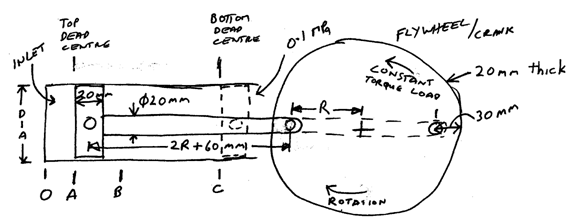## Write a computer program using matlab, MATLAB Programming

Assignment Help:

From A to B the inlet valve is open and the steam pressure increases linearly from 0.1000 MPa absolute to 15.00 MPa absolute, 1000°C.

The inlet valve closes and from B to C the steam expands isentropically and adiabatically to the end of the stroke, where the steam pressure is 0.1000 MPa.

The pressure on the crank side of the piston is always 0.1000 MPa.

On the return stroke the exhaust valve is open, so the pressure on both sides of the piston is 0.1000 MPa.

The distance from A to B is 10 + DD/10 mm.

At top dead centre (top of stroke) the distance OA is 10 + BB/10 mm.For the steam engine given in the above diagram determine the forces on each of the components when the engine is running at constant revolutions per minute (angular velocity at the beginning and end of each revolution is the same but varies during the revolution).  Determine these forces at increments of 1 degree of the flywheel position and plot all forces for 1 revolution of the flywheel.  Plot the angular velocity of the flywheel.  Use the steam tables for your steam calculations.

Make the following assumptions:

(a) All components are made of steel with a density of  7800 kg/m3.

(b) Assume the piston and rod are solid steel pieces (not hollow).

(c) Flywheel speed is 500 RPM when the piston is at top dead centre.

#### Find the spectrum of waveform, (a) Find the spectrum of this waveform:  x =...

(a) Find the spectrum of this waveform:  x = [ exp( -[0:1:49]/10), exp(-[50:-1:1]/10)]. Subplot only the magnitude by using the spectrum program from the class notes. (b) Using

#### Plot the input and the output of the filter on a single plot, An FIR filter...

An FIR filter has coefficients b = [ 1.0000   -0.6387    1.0214    0.8210   -0.7470    1.0920 ] (a) Find H(z) for the filter and plot its frequency response (magnitude and phase

#### Physics, how do you make a program for a parachute man falling to determine...

how do you make a program for a parachute man falling to determine his terminal velocity, having in consideration the drag force (cv^2). I have the formula to be v(i+1)=v(i)*delta(

#### Image processing, i am doing my project on matlab and the topic of my proje...

i am doing my project on matlab and the topic of my project is invisible watermarking.How we can extract all pixel values of image in binary form.

#### Help with MATLAB, I have couple questions in MATLAB I need help in

I have couple questions in MATLAB I need help in

#### String, how to convert char array to a string cell

how to convert char array to a string cell

#### Basic algebra, how to find absolute value of a number

how to find absolute value of a number

#### Iterative root fi nding method, The secant method is an iterative root ndi...

The secant method is an iterative root nding method that is super-linear. The method has the advantage that it is faster than linear methods and does not require knowledge of the

#### Subpixel boundary., could you help me object

could you help me object

#### Operators, Operators: There are in general two types of operators: una...

Operators: There are in general two types of operators: unary operators that operate on a single value or operand; and binary operators, that operate on two values or operands